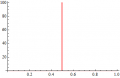# The time required for the current to grow to

#### ironasona

Joined Nov 12, 2020
53
How do i find the '' The time required for the current to grow to ......Amps''

for example lets say i need to find the The time required for the current to grow to 2.5Amps
R=33 Ohms
L=3.3H

would i do 3.03(1-e^33/3.3)? is this right ? or have i done this wrong

#### djsfantasi

Joined Apr 11, 2010
9,124
Excuse me, but “the current to grow to” what in where? With the information you’ve given, the answer could be infinity because there is no circuit!

#### Papabravo

Joined Feb 24, 2006
20,583
If you have an R, and an L, and a voltage source then the current will grow exponentially to to a final value defined by the resistor and any parasitic DC resistance in the inductor.
Have you done it correctly -- I can't tell. The numbers by themselves don't mean anything without units. For example: what does 3.03 refer to?

#### RBR1317

Joined Nov 13, 2010
712

#### Papabravo

Joined Feb 24, 2006
20,583
The answer depends critically on the kind of source you use. Here is a set of plots of an RL circuit with the sources of 20, 50, 82.5 & 100 Volts. A summary of the results:
1. For Vcc < 82.5 you can never get to 2.5 Amps
2. For Vcc = 82.5V you will approach 2.5 volts asymptotically. That means you will never get there.
3. For Vcc > 82.5 volts you will get there in a finite amount of time which you can calculate from the formula
BTW 33 Ω × 2.5 Amperes = 82.5 Volts

#### RBR1317

Joined Nov 13, 2010
712
It seems this is beginning to address the area of switched RL & RC circuits where one must consider the state of the circuit just before the switching event and the state of the circuit a very long time after the switching event in order to recognize the initial value & final value of the circuit conditions.

Note that for a capacitor, the current through the capacitor can switch to a new value instantaneously but the voltage across the capacitor must follow an exponential curve from the initial to the final value.

For an inductor, the voltage across the inductor can switch to a new value instantaneously but the current through the inductor must follow an exponential curve from the initial to the final value.

So for switched RL & RC circuit behavior, always determine the following:

a. Initial Value
b. Final Value (i.e., at t = ∞)
c. Time Constant
d. Rising or Falling Exponential Curve {(Final Value)-(Initial Value)}

#### Papabravo

Joined Feb 24, 2006
20,583
It seems this is beginning to address the area of switched RL & RC circuits where one must consider the state of the circuit just before the switching event and the state of the circuit a very long time after the switching event in order to recognize the initial value & final value of the circuit conditions.

Note that for a capacitor, the current through the capacitor can switch to a new value instantaneously but the voltage across the capacitor must follow an exponential curve from the initial to the final value.

For an inductor, the voltage across the inductor can switch to a new value instantaneously but the current through the inductor must follow an exponential curve from the initial to the final value.

So for switched RL & RC circuit behavior, always determine the following:

a. Initial Value
b. Final Value (i.e., at t = ∞)
c. Time Constant
d. Rising or Falling Exponential Curve {(Final Value)-(Initial Value)}
The voltage across an inductor cannot change instantaneously, it is limited by the value of di/dt. It can be large, but it cannot be infinite.

#### RBR1317

Joined Nov 13, 2010
712
The voltage across an inductor cannot change instantaneously
It certainly can in a switched circuit. However, as current begins to flow in the inductor, the coil voltage will fall to zero according to the circuit time constant.

#### Papabravo

Joined Feb 24, 2006
20,583
Are you saying that the switch can create a di/dt that is infinite, as opposed to just large?

#### RBR1317

Joined Nov 13, 2010
712
No. The switch creates a dv/dt that is infinite; the di/dt is zero. At t=0+ the coil voltage is whatever has been applied by the external switched circuit, and begins to drop with a slope controlled by the L/R time constant as the inductor current begins to increase.

#### Papabravo

Joined Feb 24, 2006
20,583
No. The switch creates a dv/dt that is infinite; the di/dt is zero. At t=0+ the coil voltage is whatever has been applied by the external switched circuit, and begins to drop with a slope controlled by the L/R time constant as the inductor current begins to increase.
When it comes to real components it can be hard to distinguish large from infinite. I'll continue with my reality and you continue with yours.

#### RBR1317

Joined Nov 13, 2010
712
When it comes to real components it can be hard to distinguish large from infinite. I'll continue with my reality and you continue with yours.
When it comes to circuit analysis the usual rule is to use ideal components unless the problem specifically states how much of reality to include in the analytical result. There is no "my reality"; there is only the problem statement.

#### The Electrician

Joined Oct 9, 2007
2,952
The voltage across an inductor cannot change instantaneously,
You're talking about the "change" in voltage across an inductor. This is dv/dt.

it is limited by the value of di/dt. It can be large, but it cannot be infinite.
The change in voltage (dv/dt) is not controlled by di/dt; the magnitude of the voltage (that is just v) is controlled by di/dt.

The constitutive equation for an inductor is v = L*di/dt.

Imagine you have an ideal 1 henry inductor with initially zero current in it. Now suddenly apply 10 volts (dv/dt = ∞). At t = 0+ the current will begin to ramp up with a constant slope. That slope has a value of v/L and multiplying the slope (di/dt) by the value of L gives the applied voltage. Applying the voltage very quickly (dv/dt = ∞) does not cause the current in the inductor to increase more rapidly. The rate at which the inductor current increases is only proportional to the magnitude of the applied voltage, not how quickly it is applied.

To make the inductor current increase more rapidly one must apply a higher voltage (v = big)

#### Papabravo

Joined Feb 24, 2006
20,583
...

The constitutive equation for an inductor is v = L*di/dt.

...
Fine, if:
$v\;=\;L\frac{di}{dt},\;\; \text{then}$
$\frac{dv}{dt}\;=\;\frac{d}{dt} \left (L\frac{di}{dt}\right)\;=\;L\frac{d^2i}{dt^2}$

You still can't get dv/dt to be infinite

#### RBR1317

Joined Nov 13, 2010
712
You still can't get dv/dt to be infinite
You can if you don't ignore the obvious. "dv" is a finite quantity equal to the magnitude of the switched voltage. "dt" is equal to the duration of the switching event, which is zero. Division by zero yields infinity.

#### The Electrician

Joined Oct 9, 2007
2,952
Here is an example of the current in an ideal inductor when a voltage is applied with less than infinite speed: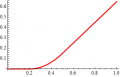Here is the voltage that was applied to get that current. This is not a step function of voltage, but rather a voltage that ramps at finite speed to its final value. This is the first derivative of the current, di/dt: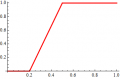Here is the first derivative of the applied voltage, dv/dt, and it's also the second derivative of the current d2i/dt2: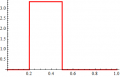#### The Electrician

Joined Oct 9, 2007
2,952
Here's what happens when the applied voltage ramps up at a higher speed. Here's the current in the inductor in this case. We don't see the slow increase to the final ramp of current this time because the voltage ramps so fast (but not infinitely fast--just fast) that the current in the inductor looks like it goes from zero to the final ramp right away: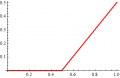Here is the voltage that was applied to get that current. This is not a step function of voltage, but rather a voltage that ramps at fast finite speed to its final value. This is the first derivative of the current, di/dt: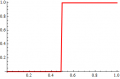Here is the first derivative of the applied voltage, dv/dt, and it's also the second derivative of the current d2i/dt2. In the limit of infinitely fast application of voltage to the inductor, this spike will become a Dirac delta function. The second derivative of an inductor current that starts as zero and then transitions to a linear ramp without a gradual transition to the ramp as shown in the previous post is an impulse, infinitely tall and infinitesimally narrow. The first derivative of a voltage which is applied infinitely fast is the same impulse.